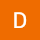Home Communities
IT Knowledge
Inspiration
Languages
EN

# Arduino - draw charts by using Serial Plotter

13 points
Created by:Noname
2430

Serial Plotter is a very useful feature of Arduino IDE for drawing linear charts. This post contains two examples that explain how to use it.

## 1. Simple example - zigzag line

A very simple example where variable y is increased from 0 to 10 by adding 1 in each iteration. If the value reaches 10, the y variable is back to 0.

Step 1. Write code below and upload a compiled program to Arduino (Sketch -> Upload).

Note: For single chart every value has to be printed in a new line (use: Serial.println() instead of Serial.print()).

``````int y = 0;

void setup() {
// enable serial communication between Arduino and PC
Serial.begin(9600);
}

void loop() {
y += 1;
if(y > 10) y = 0;

Serial.println(y);

// wait 10 ms
delay(10);
}``````

Step 2. Open Serial Plotter (Tools -> Serial Plotter). In the new window you will see a similar chart:

## 2. Three charts example

There is a possibility to draw more than only one chart at the same time. The example code bellow drawing three charts: zigzag line (y1), straight line (y2) and sinus function (y3).

Note: For three charts, three values have to be printed in a single line. Values in line have to be separated by space or tab.

Step 1. Write code below and upload a compiled program to Arduino (Sketch -> Upload).

``````int y1 = 0, y2 = 15;
double x3 = 0.0, y3;

void setup() {
// enable serial communication between Arduino and PC
Serial.begin(9600);
}

void loop() {
y1 += 1;
if(y1 > 10) y1 = 0;

y3 = sin(x3) -10.0;
x3 += 0.1;

Serial.print(y1); Serial.print("\t");
Serial.print(y2); Serial.print("\t");
Serial.println(y3);

// wait 10 ms
delay(10);
}``````

Step 2. Open Serial Plotter (Tools -> Serial Plotter). In the new window you will see a three charts:

## Alternative titles

Join to our subscribers to be up to date with content, news and offers.